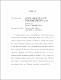## Locally symmetric spaces and the cohomology of the Weil representationWe study generalized special cycles on Hermitian locally symmetric spaces $\Gamma \backslash D$ associated to the groups $G=\mathrm{U}(p,q)$, $\mathrm{Sp}(2n,\R)$ and $\mathrm{O}^*(2n)$. These cycles are covered by symmetric spaces associated to subgroups of $G$ which are of the same type. Using the oscillator representation and the thesis of Greg Anderson (\cite{Anderson}), we show that Poincar\'e duals of these generalized special cycles can be viewed as Fourier coefficients of a theta series. This gives new cases of theta lifts from the cohomology of Hermitian locally symmetric manifolds associated to $G$ to vector-valued automorphic functions associated to the groups $G'=\mathrm{U}(m,m)$, $\mathrm{O}(m,m)$ or $\mathrm{Sp}(m,m)$ which are members of a dual pair with $G$ in the sense of Howe. The above three groups are all the groups that show up in real reductive dual pairs of type I whose symmetric spaces are of Hermitian type with the exception of $\mathrm{O}(p,2)$.